# How to Calculate Square Roots – Excel & Google Sheets

This tutorial will teach you how to calculate square roots in Excel and Google Sheets.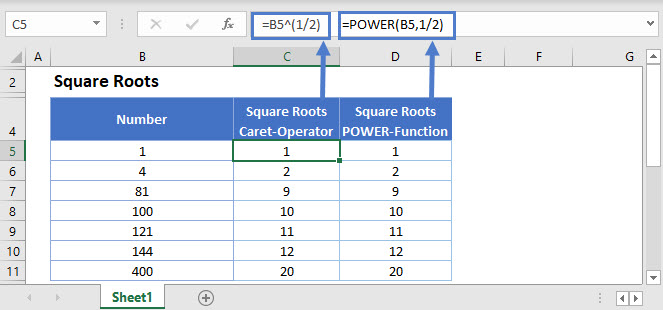## Square Root using SQRT Function

One way to find the square root of a number is using the SQRT function. Its syntax is: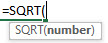``=SQRT(B3)``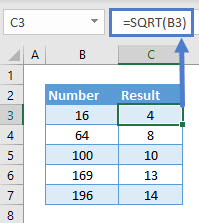To find the square root of a list of numbers drag the formula down as shown in the example.

Square Root using Exponent Operator

Another way to find the square root of a number is to use the exponent operator (^) with 1/2 as the exponential.

Let’s find the square root of a number using the exponent operator for the same example.

``=B3^(1/2)``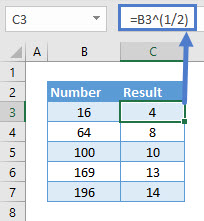Note: Make sure the exponential ‘1/2’ is in parentheses to control the order of operations.

Square Root using POWER Function

The POWER function returns the value for a given number raised to a power. Its syntax is: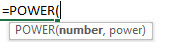Let’s find the square root of a number using the POWER function for the same example.

``=POWER(B3,1/2)``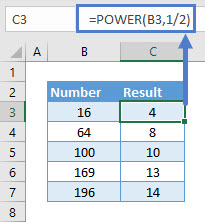Square Root of a Negative Number using ABS Function

The ABS function which returns the absolute value for any negative number can be nested in any of the three functions explained above (the SQRT function, the Exponent Operator and the POWER function) to find the square root of a negative number. Its syntax is: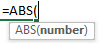Let’s find the square root of a list of negative numbers using the ABS function nested in the SQRT function.

``=SQRT(ABS(B3))``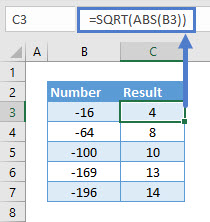The ABS function can be used in a similar manner for the other two functions (the Exponent Operator and the POWER function)

Note: Excel returns the #NUM! error if the ABS function is not used for calculating the square root of a negative number.

Note 2: Instead to take the square of a number, use 2 instead of 1/2.

## Square Root in Google Sheets

These formulas work exactly the same in Google Sheets as in Excel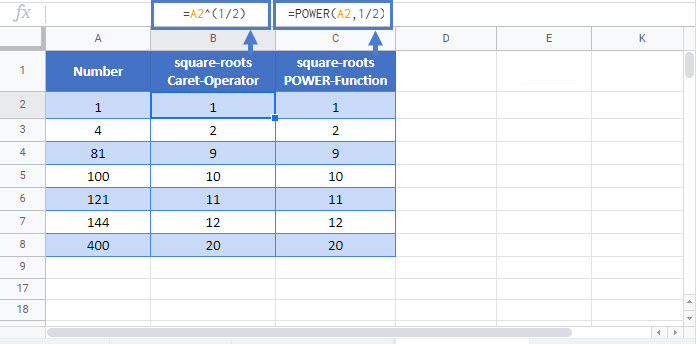### Excel Practice Worksheet

Practice Excel functions and formulas with our 100% free practice worksheets!